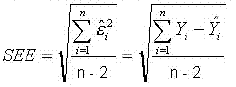### CFA Practice Question

There are 208 practice questions for this study session.

### CFA Practice Question

Which of the following statements is true with respect to the Standard Error of the Estimate (SEE)?

I. SEE measures the dispersion around the regression line.
II. SEE requires the summation of the squares of the differences between the predicted values of Y and the mean of Y.
III. SEE requires n-2 degrees of freedom.
IV. SEE will always decrease as more observations are added.

Standard Error of Estimate (SEE) may be found as follows:where Y-hati is the regression for the Y value, given the X value.

Therefore, II is false because SEE requires the summation of the squares of the differences between the observed value for Y and its value as predicted by the regression.

IV is also false because if these new observations have a greater dispersion from the regression line, then it will tend to enlarge the SEE.